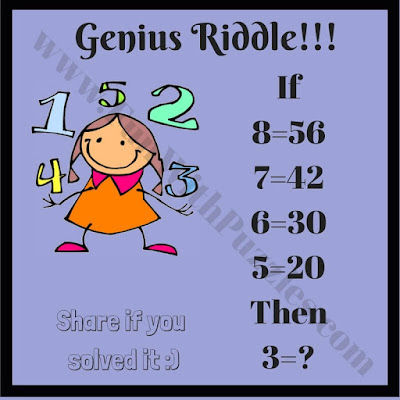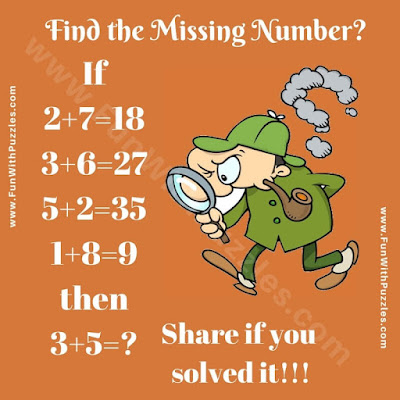There are many different types of brain teasers published on this website. Some of the brain teasers are picture brain teasers in which one has to find hidden animals in the given picture. Some are Mathematical Brain Teasers in which one has to do mathematical calculations to find the answer. Also, there are some brain teasers in which one has to count the number of given geometrical shapes in the given picture image. There are some Quick Brain Teasers too in which one has to quickly answer the given riddles. Also, there are Logical Puzzles that have to solve the logical equation.

Here are the logical puzzles which are the extension of "Interesting Brain Teasers to Challenge Your Mind".

## How to Solve Mental Ability Test Reasoning Puzzle Questions?

In this mental ability test, there are some mathematical equations that follow some logical pattern in calculating the answer. One has to find this logical pattern and then solve the last equation to find the value of the question mark.6. Can you find the value of the missing number?

Don't miss to solve similar Logical and Mathematical puzzles in which Math equations are given that follow some logical pattern. One has to find this logical pattern and has to tell which number will replace the question mark?7. Can you solve this Logical Puzzle?8. Can you solve this tough brain teaser?9. Can you find the missing Number?10. Can you solve this tough Logical Puzzle?

If you solved most of these puzzles correctly then you should definitely try Awesome Brainteasers and Simple Math Riddles.

Unknown said...

6_ ANS : 75